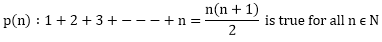×#### Thank you for registering.

One of our academic counsellors will contact you within 1 working day.

Click to Chat

1800-1023-196

+91-120-4616500

CART 0

• 0

MY CART (5)

Use Coupon: CART20 and get 20% off on all online Study Material

ITEM
DETAILS
MRP
DISCOUNT
FINAL PRICE
Total Price: Rs.

There are no items in this cart.
Continue Shopping• Complete JEE Main/Advanced Course and Test Series
• OFFERED PRICE: Rs. 15,900
• View Details

```Chapter 12: Mathematical Induction – Exercise 12.1

Mathematical Induction – Exercise 12.1 – Q.1

p(n) : n(n + 1) is even

p(3) : 3.(3 + 1) is even

Mathematical Induction – Exercise 12.1 – Q.2

p(n) : n3 + n is divisible by 3

p(3) : 33 + 3 is divisible by 3

⟹ p(3) : 30 is divisible by 3.

∴ p(3) is true

Now,

p(4) : 43 + 3 = 67 is divisible by 3

since, 67 is not divisible by 3

so, p(4) is not true

Mathematical Induction – Exercise 12.1 – Q.3

p(n) : 2n ≥ 3n

Given that p(r) is true

⟹ 2r ≥ 3r

Multiplying both the sides by 2,

2.2r  ≥ 2.3r

2r+1 ≥ 6r

≥ 3r + 3r

≥ 3 + 3r,         [since 3r ≥ 3 ⟹ 3r + 3r ≥ 3 + 3r]

2r+1 ≥ 3(r + 1)

⟹ p(r + 1) is true

Mathematical Induction – Exercise 12.1 – Q.4

Here, p(n) : n2 + n is even

Given, p(r) is true

⟹ r2 + r is even

⟹ r2+ r = 2λ  ---- (i)

Now,

(r + 1)2 + (r + 1)

= r2 + 2r + 1 + r + 1

= (r2 + r) + 2r + 2

= 2λ + 2r + 2           [Using equation (1)]

= 2(λ + r + 1)

= 2µ

⟹ (r + 1)2 + (r + 1) is even

⟹ p(r + 1) is true

Mathematical Induction – Exercise 12.1 – Q.5Mathematical Induction – Exercise 12.1 – Q.6

p(n) : n2 - n + 41 is prime

p(1) : 1 - 1 + 41 is prime

⟹ p(1) : 41 is prime

∴ p(1) is true.

p(2) : 22 - 2 + 41 is prime

⟹ p(2) : 43 is prime

∴ p(2) is true.

p(3) : 32 - 3 + 41 is prime

⟹ p(3): 47 is prime

∴ p(3) is true.

p(41): (41)2 - 41 + 41 is prime

p(41): (41)2 is prime

⟹ p(41) is not true

```### Course Features

• 728 Video Lectures
• Revision Notes
• Previous Year Papers
• Mind Map
• Study Planner
• NCERT Solutions
• Discussion Forum
• Test paper with Video Solution